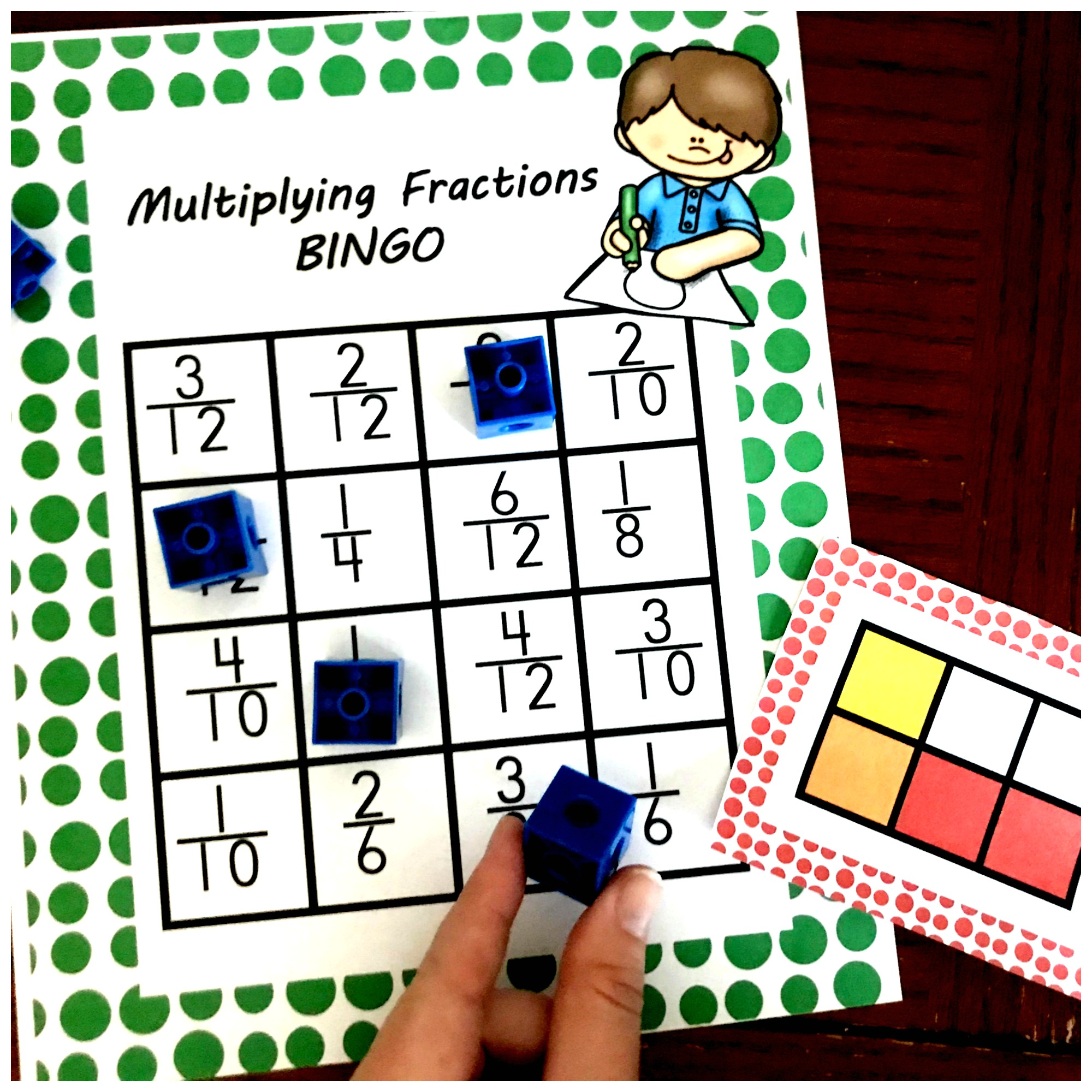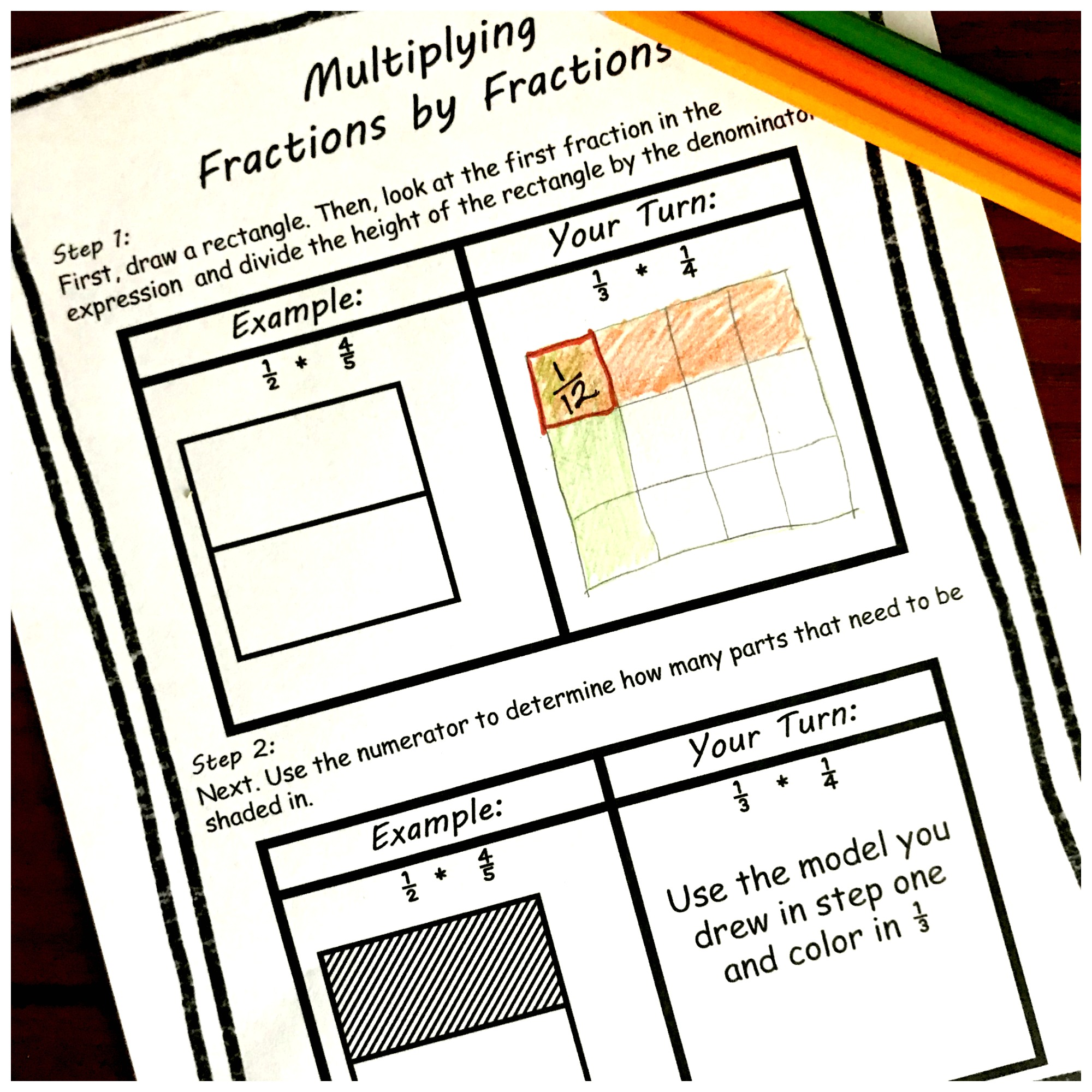Home » Posts tagged "multiplying fractions"

## 3 Cut and Paste Worksheets For Multiplying Fractions PracticeThese three cut and paste worksheets are perfect for multiplying fractions practice. It is a fun way to use area models to solve fraction by fraction problems. We get to continue our focus on multiplying fractions by fractions today!! We have already had so much...

## Here’s a Multiplying Fractions Game That’s Perfect for Extra PracticeThis free multiplying fractions game is a fun way to practice this skill. Children solve expressions and area models and then find the answer on the BINGO card. Yes, multiplying fractions by fractions is an upper elementary skill, but guess what. These big kiddos enjoy...